# Multi-Label Classification -- Metrics & Confusion Matrix Mismatch

I’ve setup multi-label classification on a private dataset. While the learning rate finder and the metrics show that the model is training well, the confusion matrix tells a different story (as do qualitative checks on model performance).

# Dataset Summary

### Constructing the DataBunch

I’ve removed the code that constructs the DataFrame with the filenames with classs for brevity.

``````lls = LabelLists(path  = '/',
train = ImageList.from_df(df_train, path='/'),
valid = ImageList.from_df(df_valid, path='/'))

data_lighting = (lls
.label_from_df(label_delim='_')
.transform(tfms=get_transforms(),
size=(224,224),
resize_method=ResizeMethod.SQUISH)
.databunch(bs=64)
.normalize(imagenet_stats))
``````

### Train + Val Distribution

``````data_lighting.c             # ==    8

len(data_lighting.train_ds) # == 3660
len(data_lighting.valid_ds) # ==  900
``````
``````vc = pd.value_counts(data_lighting.train_ds.y)
pd.DataFrame(vc, columns=['Frequency'])
``````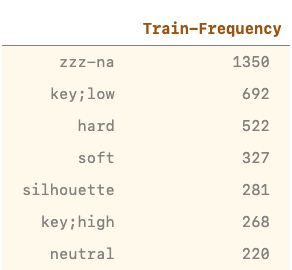``````vc = pd.value_counts(data_lighting.valid_ds.y)
pd.DataFrame(vc, columns=['Frequency'])
``````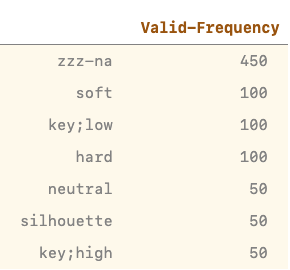# Training

### Model Setup

``````acc_02 = partial(accuracy_thresh, thresh=0.2)
f_score = partial(fbeta, thresh=0.2)

learn = cnn_learner(data_lighting, models.mobilenet_v2,
metrics=[acc_02, f_score],
path = 'home/rahul/tmp',
callback_fns=partial(SaveModelCallback, monitor='fbeta'))
``````

### LR Find + One Cycle Training

``````learn.lr_find()
learn.recorder.plot()
``````

``````learn.fit_one_cycle(5, slice(1e-2))
``````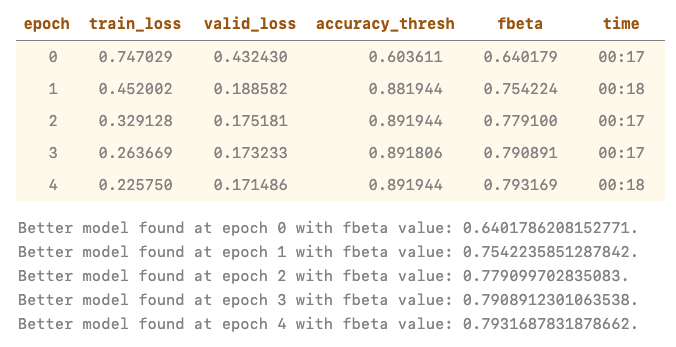# Confusion Matrix

``````interp = ClassificationInterpretation.from_learner(learn)
interp.plot_confusion_matrix()
``````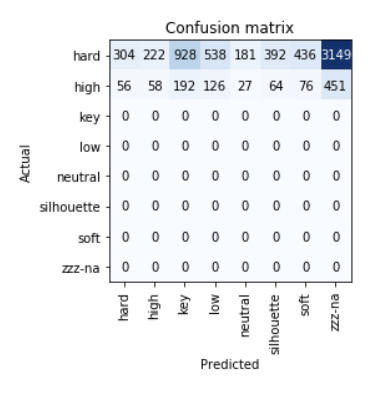## Questions

• The model is predicting everything as `hard` or `high` while the metrics tell a very different story. Is this because of the `thresh` values? (I think not)

• The number of validation samples is 900 and training samples 3660. As per the confusion matrix, there’s way more samples than both of these combined. What’s going on here?

• When training using the exact same data as a single-class classification problem, which reduces the no. of labels from 8 to 7, the model trains as expected and the confusion matrix makes sense too

(PS – the label names are different because of how I constructed the dataset, but the dataset is the same)

##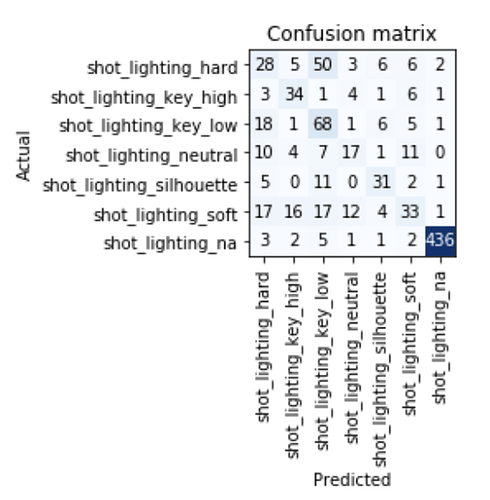Screenshot 2020-03-07 at 11.45.19 AM580×591 98.9 KB

Thank you!

I think we’re going to need to see all your code on this one. You have more data points being reported in your matrix than exists in your dataset from your screenshot.

Also those na’s in your validation metrics don’t look right either.

Thanks for respondingThe code used to create the `DataFrames` being passed into `LabelLists` is rather long and clunky. Will it be more helpful if I shared `.csv`s of `df_train` and `df_valid` ?

@wgpubs I’ve uploaded the notebook here:

Note that it takes longer than the average webpage to load completely.

Would love to hear your thoughts.

I’ll look at this today or tomorrow (struggling with a severe case of vertigo since last Sunday so it may take a day or two to get back to you)

Ohh. Get some rest, I hope you feel better soon!

No rush with this stuff, thanks a lot for your time.

So …

The confusion matrix seems to indicate that all your examples are only “N/A” and “Hard” … which is obviously not the case based on what I’m seeing in your `show_batch` call. The reason is that the confusion matrix doesn’t know how to handle multi-label intrinsically.

What you need to do is create a confusion matrix for each label … a confusion matrix per class. (see sklearn multi-label confusion matrix)

So your model is probably fine. Just remember that Confusion Matrices are for Multiclassification2 Likes

Thanks, that helps a lot.

umm… you mean single-label classification right?

That is what multiclassification is (confusing name, don’t blame me)

2 Likes

@wgpubs haha that is a confusing name…

I’m curious to hear your thoughts on another problem I’m tackling: Creating Two Models With A Common Feature Extractor